Скачать презентацию The Existential Graphs Project Rensselaer Reasoning Group September

18ba8c307314f68a48692b843c677851.ppt

• Количество слайдов: 28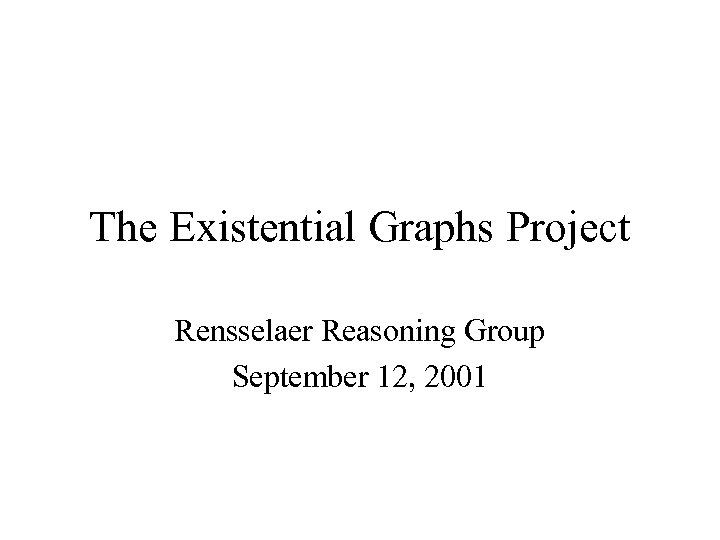The Existential Graphs Project Rensselaer Reasoning Group September 12, 2001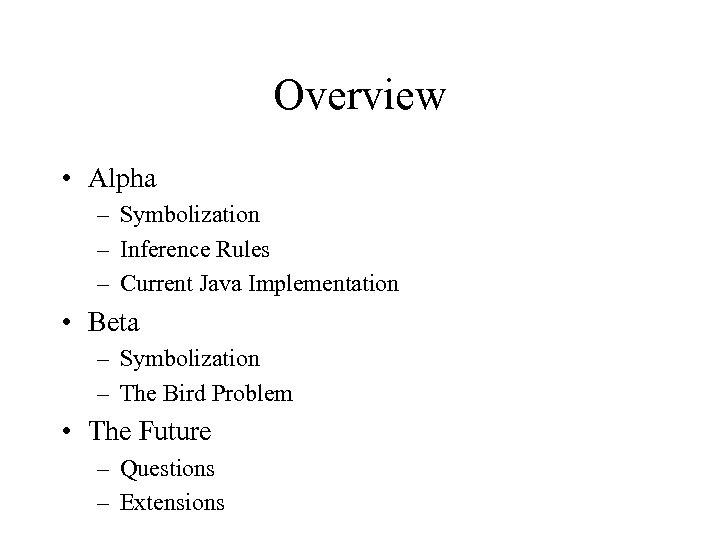Overview • Alpha – Symbolization – Inference Rules – Current Java Implementation • Beta – Symbolization – The Bird Problem • The Future – Questions – Extensions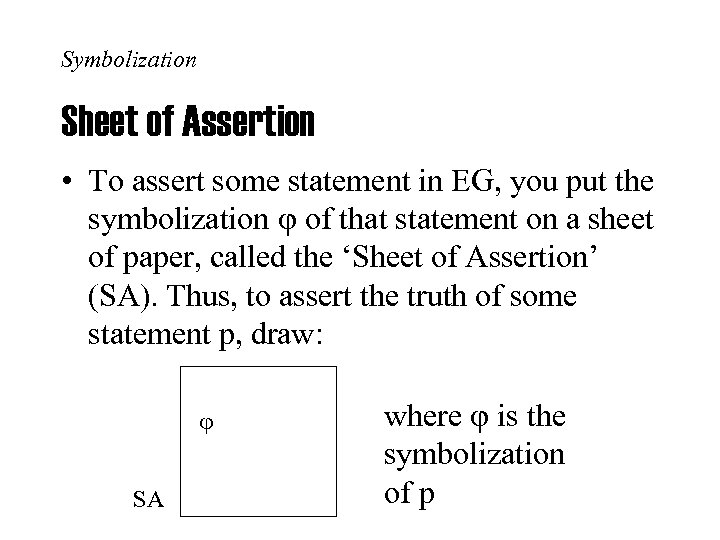Symbolization Sheet of Assertion • To assert some statement in EG, you put the symbolization of that statement on a sheet of paper, called the ‘Sheet of Assertion’ (SA). Thus, to assert the truth of some statement p, draw: SA where is the symbolization of p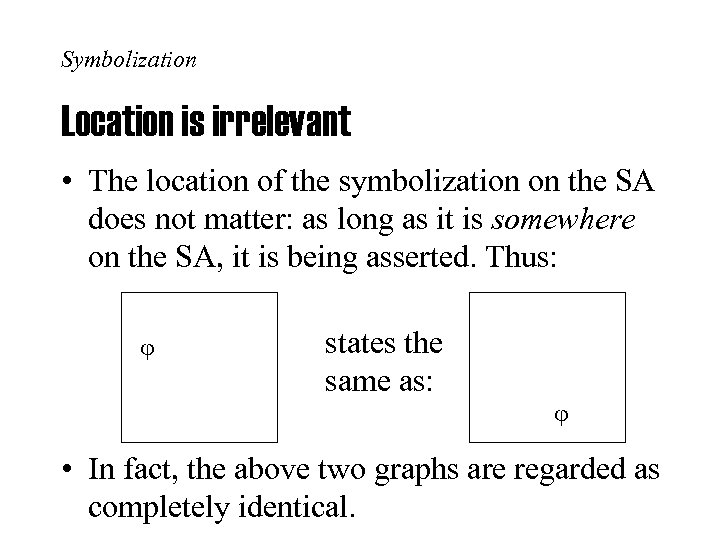Symbolization Location is irrelevant • The location of the symbolization on the SA does not matter: as long as it is somewhere on the SA, it is being asserted. Thus: states the same as: • In fact, the above two graphs are regarded as completely identical.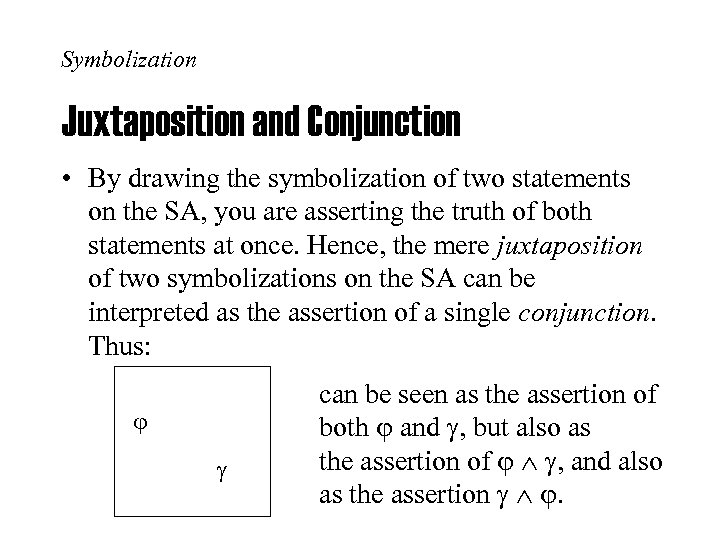Symbolization Juxtaposition and Conjunction • By drawing the symbolization of two statements on the SA, you are asserting the truth of both statements at once. Hence, the mere juxtaposition of two symbolizations on the SA can be interpreted as the assertion of a single conjunction. Thus: can be seen as the assertion of both and , but also as the assertion of , and also as the assertion .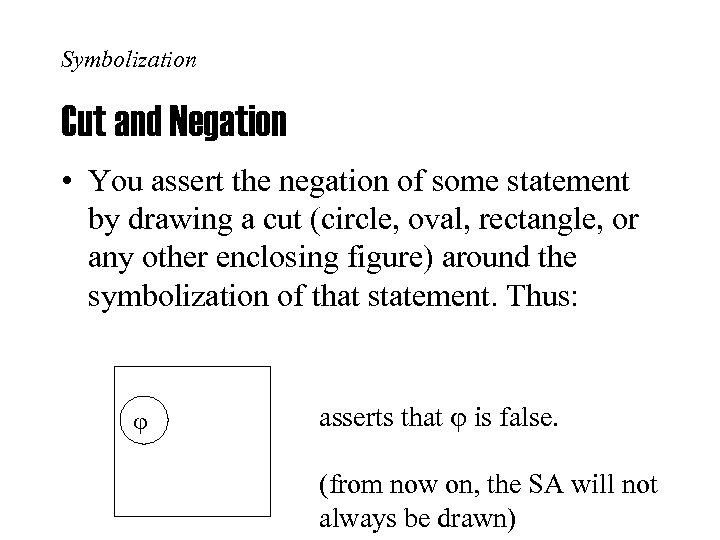Symbolization Cut and Negation • You assert the negation of some statement by drawing a cut (circle, oval, rectangle, or any other enclosing figure) around the symbolization of that statement. Thus: asserts that is false. (from now on, the SA will not always be drawn)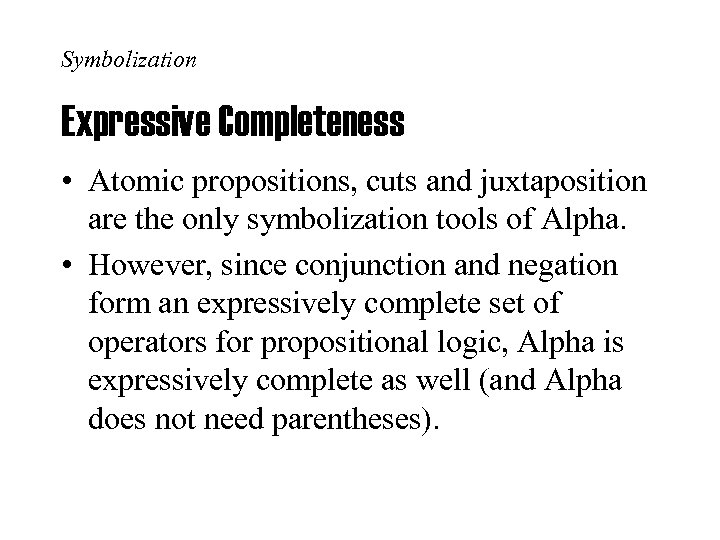Symbolization Expressive Completeness • Atomic propositions, cuts and juxtaposition are the only symbolization tools of Alpha. • However, since conjunction and negation form an expressively complete set of operators for propositional logic, Alpha is expressively complete as well (and Alpha does not need parentheses).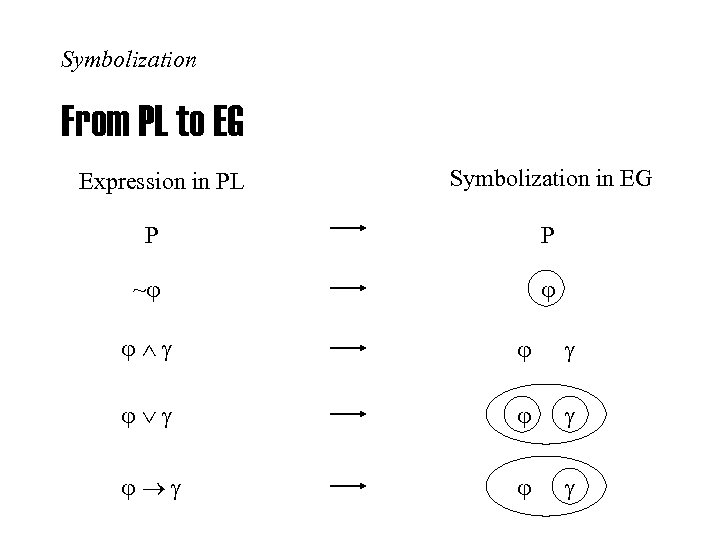Symbolization From PL to EG Expression in PL Symbolization in EG P P ~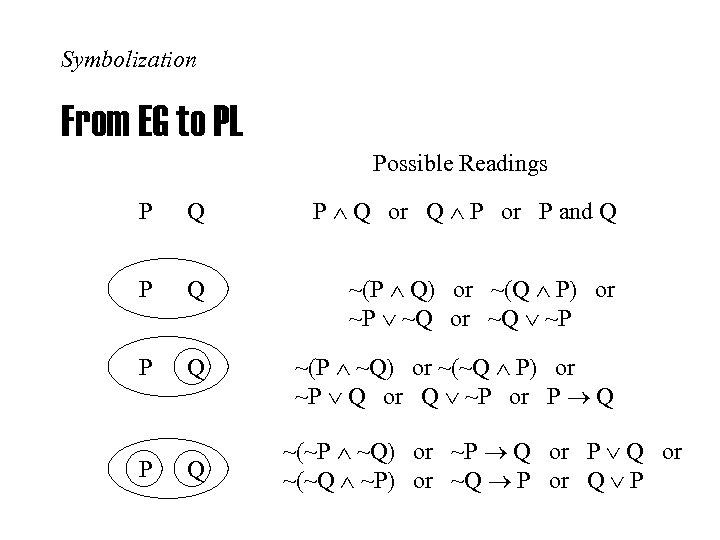Symbolization From EG to PL Possible Readings P Q P Q or Q P or P and Q P Q ~(P Q) or ~(Q P) or ~P ~Q or ~Q ~P P Q ~(P ~Q) or ~(~Q P) or ~P Q or Q ~P or P Q P Q ~(~P ~Q) or ~P Q or ~(~Q ~P) or ~Q P or Q P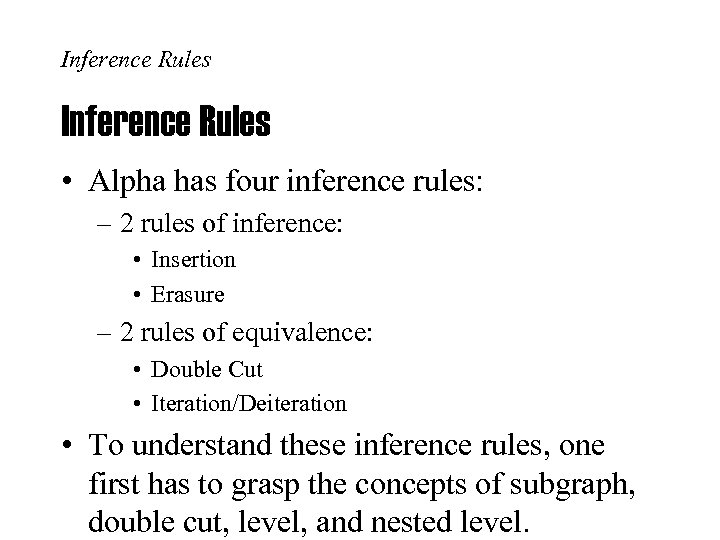Inference Rules • Alpha has four inference rules: – 2 rules of inference: • Insertion • Erasure – 2 rules of equivalence: • Double Cut • Iteration/Deiteration • To understand these inference rules, one first has to grasp the concepts of subgraph, double cut, level, and nested level.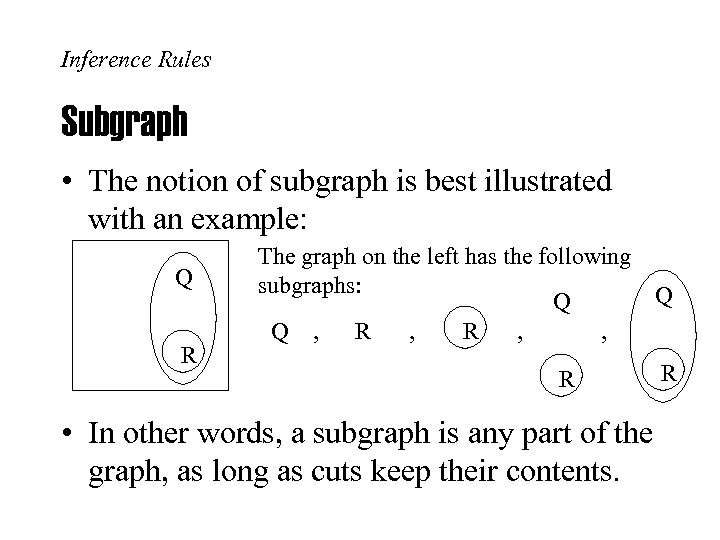Inference Rules Subgraph • The notion of subgraph is best illustrated with an example: Q R The graph on the left has the following subgraphs: Q Q Q , R , , R • In other words, a subgraph is any part of the graph, as long as cuts keep their contents. R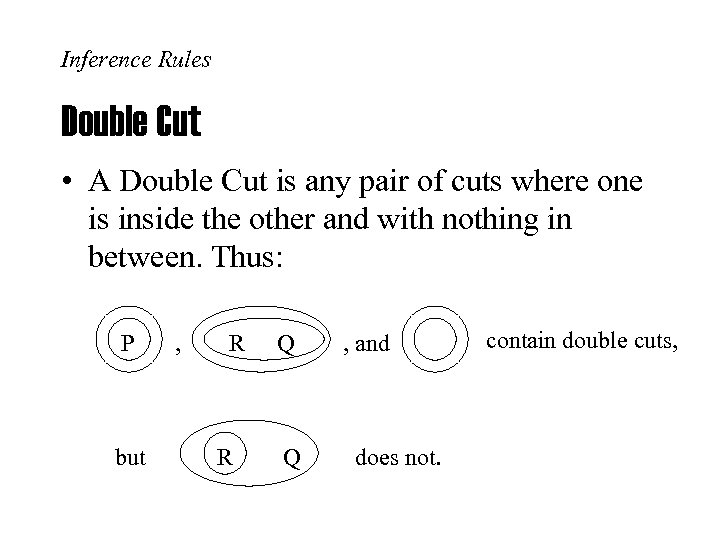Inference Rules Double Cut • A Double Cut is any pair of cuts where one is inside the other and with nothing in between. Thus: P but , R R Q Q , and does not. contain double cuts,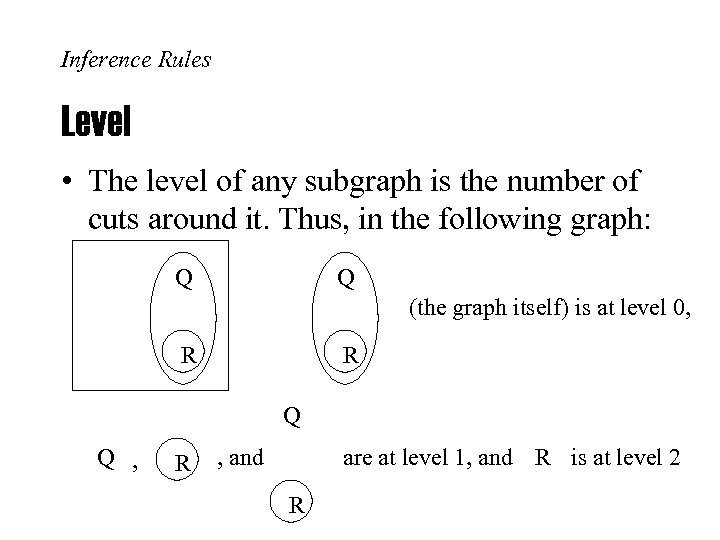Inference Rules Level • The level of any subgraph is the number of cuts around it. Thus, in the following graph: Q Q (the graph itself) is at level 0, R R Q Q , R , and are at level 1, and R is at level 2 R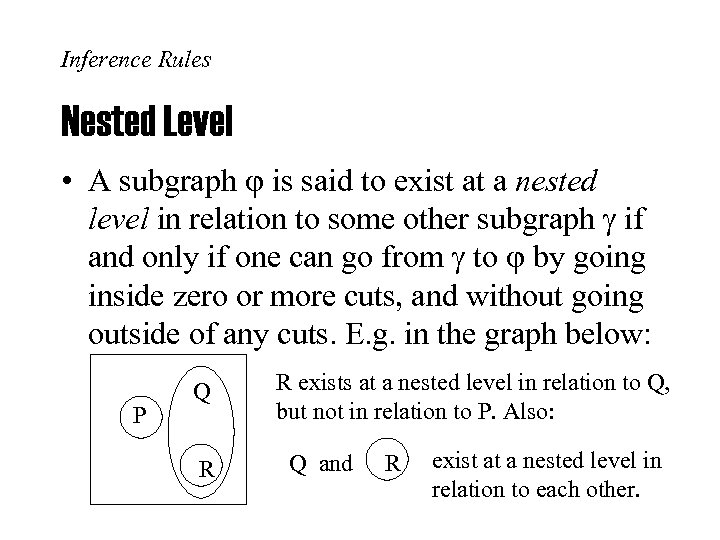Inference Rules Nested Level • A subgraph is said to exist at a nested level in relation to some other subgraph if and only if one can go from to by going inside zero or more cuts, and without going outside of any cuts. E. g. in the graph below: P Q R R exists at a nested level in relation to Q, but not in relation to P. Also: Q and R exist at a nested level in relation to each other.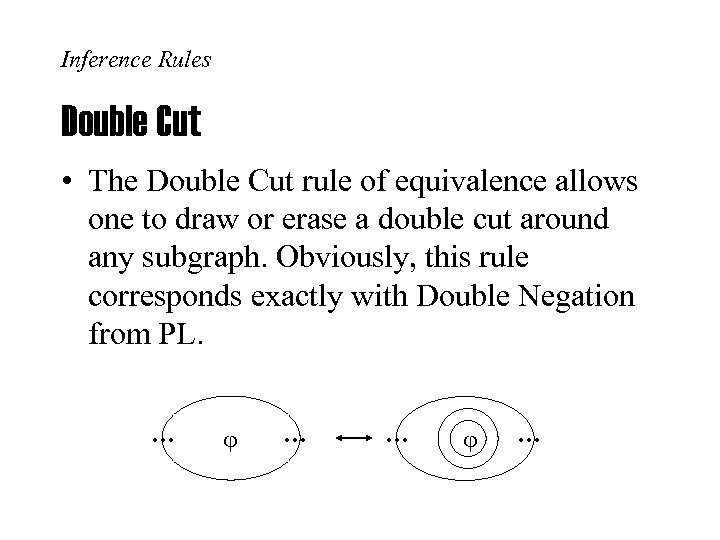Inference Rules Double Cut • The Double Cut rule of equivalence allows one to draw or erase a double cut around any subgraph. Obviously, this rule corresponds exactly with Double Negation from PL.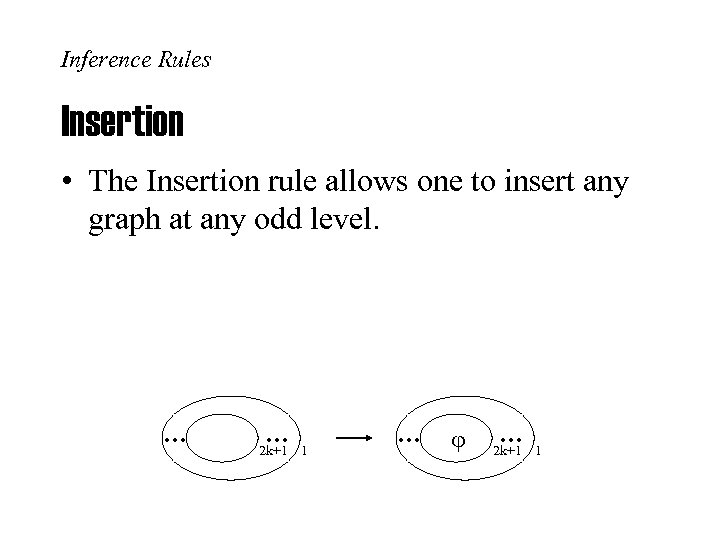Inference Rules Insertion • The Insertion rule allows one to insert any graph at any odd level. 2 k+1 1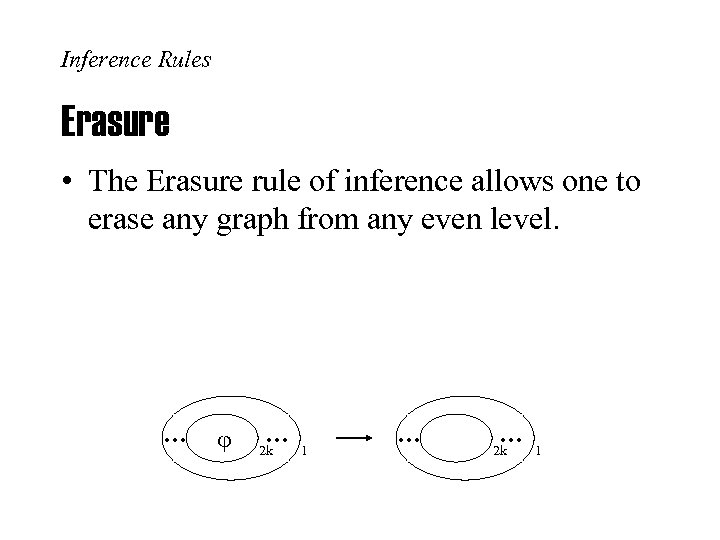Inference Rules Erasure • The Erasure rule of inference allows one to erase any graph from any even level. 2 k 1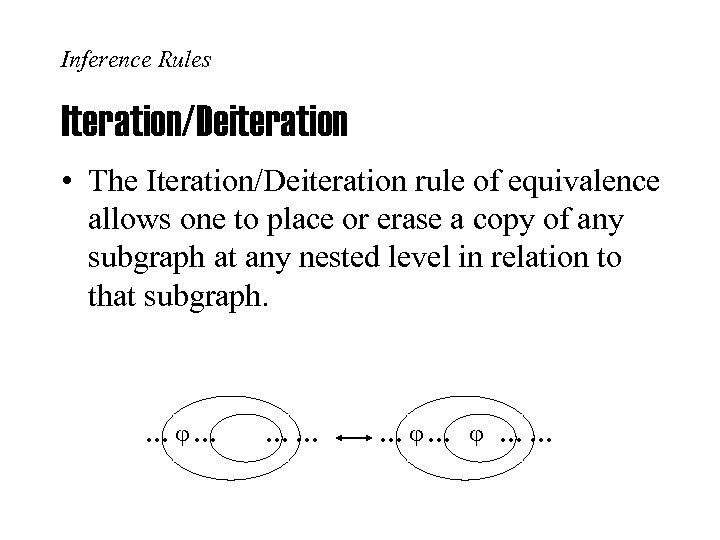Inference Rules Iteration/Deiteration • The Iteration/Deiteration rule of equivalence allows one to place or erase a copy of any subgraph at any nested level in relation to that subgraph.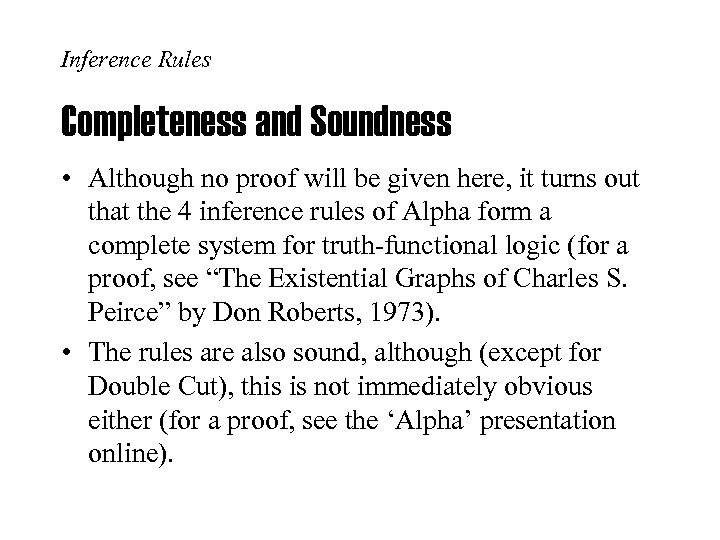Inference Rules Completeness and Soundness • Although no proof will be given here, it turns out that the 4 inference rules of Alpha form a complete system for truth-functional logic (for a proof, see “The Existential Graphs of Charles S. Peirce” by Don Roberts, 1973). • The rules are also sound, although (except for Double Cut), this is not immediately obvious either (for a proof, see the ‘Alpha’ presentation online).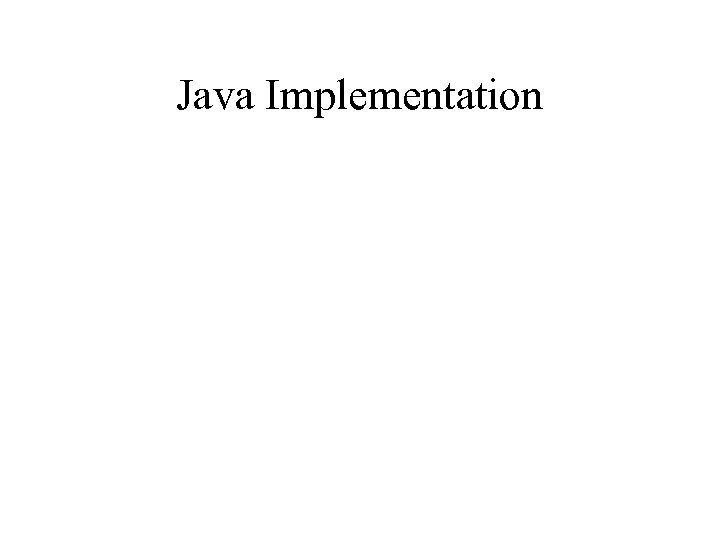Java Implementation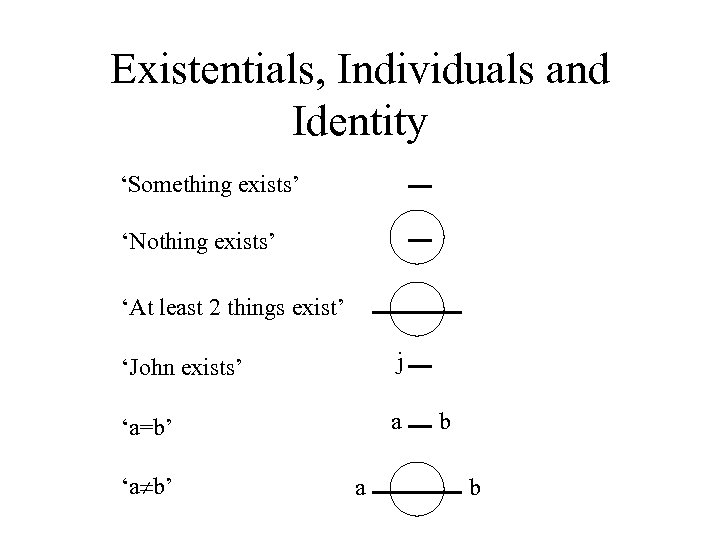Existentials, Individuals and Identity ‘Something exists’ ‘Nothing exists’ ‘At least 2 things exist’ ‘John exists’ j ‘a=b’ a ‘a b’ a b b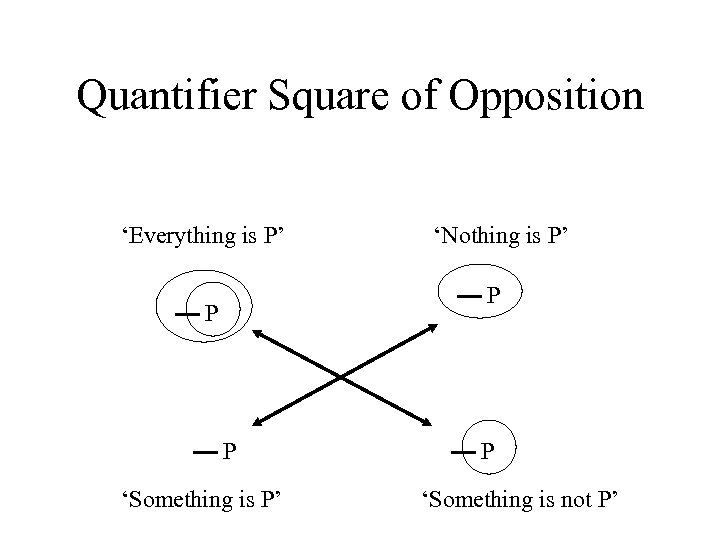Quantifier Square of Opposition ‘Everything is P’ ‘Nothing is P’ P P P ‘Something is P’ P ‘Something is not P’Traditional Square of Opposition ‘Every P is Q’ P P Q ‘No P is Q’ P P Q ‘Some P is Q’ Q Q ‘Some P is not Q’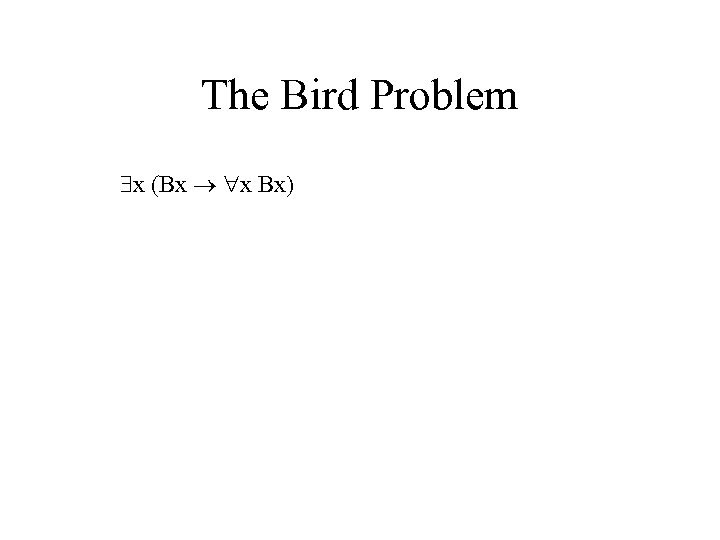The Bird Problem x (Bx x Bx)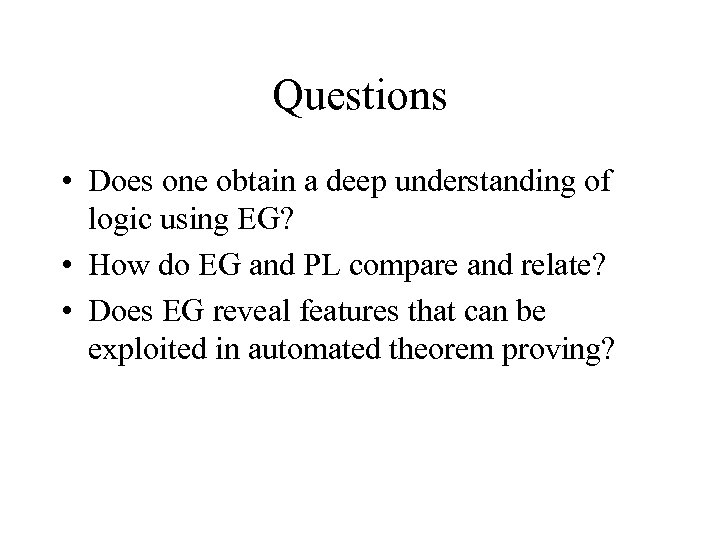Questions • Does one obtain a deep understanding of logic using EG? • How do EG and PL compare and relate? • Does EG reveal features that can be exploited in automated theorem proving?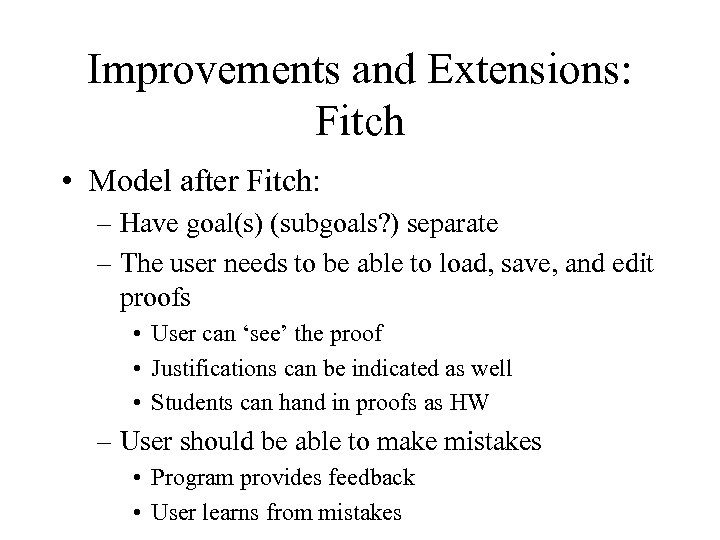Improvements and Extensions: Fitch • Model after Fitch: – Have goal(s) (subgoals? ) separate – The user needs to be able to load, save, and edit proofs • User can ‘see’ the proof • Justifications can be indicated as well • Students can hand in proofs as HW – User should be able to make mistakes • Program provides feedback • User learns from mistakes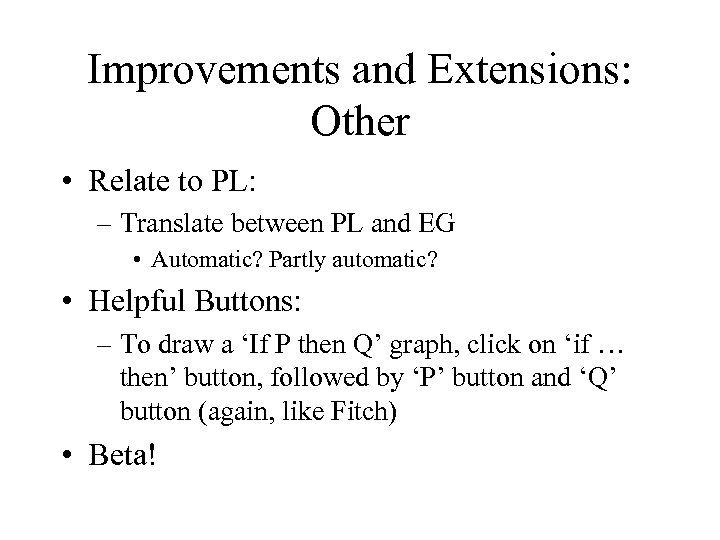Improvements and Extensions: Other • Relate to PL: – Translate between PL and EG • Automatic? Partly automatic? • Helpful Buttons: – To draw a ‘If P then Q’ graph, click on ‘if … then’ button, followed by ‘P’ button and ‘Q’ button (again, like Fitch) • Beta!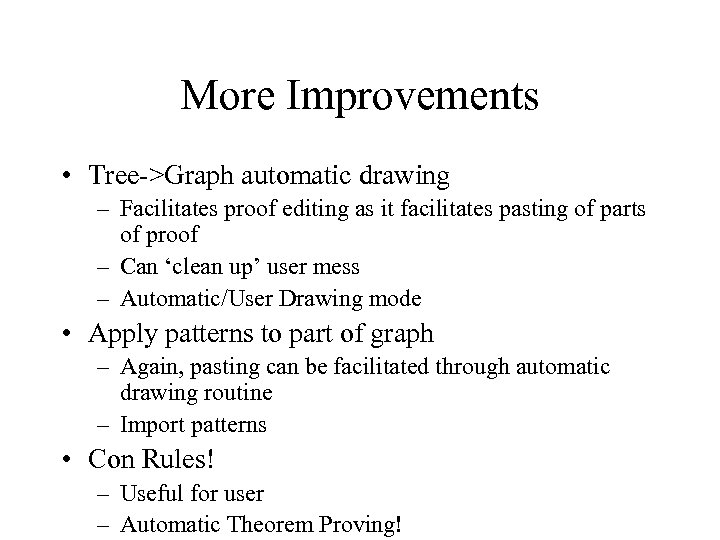More Improvements • Tree->Graph automatic drawing – Facilitates proof editing as it facilitates pasting of parts of proof – Can ‘clean up’ user mess – Automatic/User Drawing mode • Apply patterns to part of graph – Again, pasting can be facilitated through automatic drawing routine – Import patterns • Con Rules! – Useful for user – Automatic Theorem Proving!